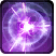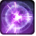# Shattering WaveConditions

Used by

## Related effects, buffs and debuffs

Please click on an effect below to view its details.

• [hidden] [does not expire]Effect #1

 Slot: Debuff Duration: passive Tick rate: does not tick # occurrences: 0 Conditions: Can only by called by other effects Target overrides: [target override] Aoe Sphere
• On Apply

Perform the following actions:

• Immobilize and prevent rotation
• [hidden] [3s]Effect #2

 Slot: Debuff Duration: 3s Tick rate: 0.2s # occurrences: 1 Tags: tag.​abl.​utility.​is_aoe Conditions: Can only by called by other effects
• On Apply

Perform the following actions:

• Play appearance epp . daily_area . ossus . story . empire . boss . boss_2 . shattering_wave, dependent on calling effect
• When effect ticks the 1th time
• [target override]

Only when the following conditions are met:

• <
• If PRIMARY TARGET is alive
AND
• If target is an enemy
>

Perform the following actions:

• Spell Damage
- Unknown (609) = (bool) false
- Damage Type = (int) 2
- Slot = (int) 1
- Spell Type = (int) 3
- Level Cap = (int) 0
- Amount Max = (float) 0
- Amount Min = (float) 0
- Amount Percent = (float) 0
- Threat Percent = (float) 0
- Standard Health Percent Max = (float) 0.23
- Standard Health Percent Min = (float) 0.23
- Amount Modifier Percent = (float) 0
- Coefficient = (float) 0
- Health Steal Percentage = (float) 0
• Add effect #3 to TARGET from TARGET, Combat Math Only
• Play appearance epp . daily_area . ossus . story . empire . boss . boss_2 . shattering_wave_impact, dependent on calling effect
• When effect ticks the 2th time
• [target override]

Only when the following conditions are met:

• <
• If PRIMARY TARGET is alive
AND
• If target is an enemy
AND
• If CASTER is positioned 5.2m to 10m to center of TARGET
>

Perform the following actions:

• Spell Damage
- Unknown (609) = (bool) false
- Damage Type = (int) 2
- Slot = (int) 1
- Spell Type = (int) 3
- Level Cap = (int) 0
- Amount Max = (float) 0
- Amount Min = (float) 0
- Amount Percent = (float) 0
- Threat Percent = (float) 0
- Standard Health Percent Max = (float) 0.23
- Standard Health Percent Min = (float) 0.23
- Amount Modifier Percent = (float) 0
- Coefficient = (float) 0
- Health Steal Percentage = (float) 0
• Add effect #3 to TARGET from TARGET, Combat Math Only
• Play appearance epp . daily_area . ossus . story . empire . boss . boss_2 . shattering_wave_impact, dependent on calling effect
• When effect ticks the 3th time
• [target override]

Only when the following conditions are met:

• <
• If CASTER is positioned 10.1m to 15m to center of TARGET
AND
• If PRIMARY TARGET is alive
AND
• If target is an enemy
>

Perform the following actions:

• Spell Damage
- Unknown (609) = (bool) false
- Damage Type = (int) 2
- Slot = (int) 1
- Spell Type = (int) 3
- Level Cap = (int) 0
- Amount Max = (float) 0
- Amount Min = (float) 0
- Amount Percent = (float) 0
- Threat Percent = (float) 0
- Standard Health Percent Max = (float) 0.23
- Standard Health Percent Min = (float) 0.23
- Amount Modifier Percent = (float) 0
- Coefficient = (float) 0
- Health Steal Percentage = (float) 0
• Add effect #3 to TARGET from TARGET, Combat Math Only
• Play appearance epp . daily_area . ossus . story . empire . boss . boss_2 . shattering_wave_impact, dependent on calling effect
• When effect ticks the 4th time
• [target override]

Only when the following conditions are met:

• <
• If PRIMARY TARGET is alive
AND
• If target is an enemy
AND
• If CASTER is positioned 15.1m to 20m to center of TARGET
>

Perform the following actions:

• Spell Damage
- Unknown (609) = (bool) false
- Damage Type = (int) 2
- Slot = (int) 1
- Spell Type = (int) 3
- Level Cap = (int) 0
- Amount Max = (float) 0
- Amount Min = (float) 0
- Amount Percent = (float) 0
- Threat Percent = (float) 0
- Standard Health Percent Max = (float) 0.23
- Standard Health Percent Min = (float) 0.23
- Amount Modifier Percent = (float) 0
- Coefficient = (float) 0
- Health Steal Percentage = (float) 0
• Add effect #3 to TARGET from TARGET, Combat Math Only
• Play appearance epp . daily_area . ossus . story . empire . boss . boss_2 . shattering_wave_impact, dependent on calling effect
• When effect ticks the 5th time
• [target override]

Only when the following conditions are met:

• <
• If PRIMARY TARGET is alive
AND
• If target is an enemy
AND
• If CASTER is positioned 20.1m to 25m to center of TARGET
>

Perform the following actions:

• Spell Damage
- Unknown (609) = (bool) false
- Damage Type = (int) 2
- Slot = (int) 1
- Spell Type = (int) 3
- Level Cap = (int) 0
- Amount Max = (float) 0
- Amount Min = (float) 0
- Amount Percent = (float) 0
- Threat Percent = (float) 0
- Standard Health Percent Max = (float) 0.23
- Standard Health Percent Min = (float) 0.23
- Amount Modifier Percent = (float) 0
- Coefficient = (float) 0
- Health Steal Percentage = (float) 0
• Add effect #3 to TARGET from TARGET, Combat Math Only
• Play appearance epp . daily_area . ossus . story . empire . boss . boss_2 . shattering_wave_impact, dependent on calling effect
• When effect ticks the 6th time
• [target override]

Only when the following conditions are met:

• <
• If PRIMARY TARGET is alive
AND
• If target is an enemy
AND
• If CASTER is positioned 25.1m to 30m to center of TARGET
>

Perform the following actions:

• Spell Damage
- Unknown (609) = (bool) false
- Damage Type = (int) 2
- Slot = (int) 1
- Spell Type = (int) 3
- Level Cap = (int) 0
- Amount Max = (float) 0
- Amount Min = (float) 0
- Amount Percent = (float) 0
- Threat Percent = (float) 0
- Standard Health Percent Max = (float) 0.23
- Standard Health Percent Min = (float) 0.23
- Amount Modifier Percent = (float) 0
- Coefficient = (float) 0
- Health Steal Percentage = (float) 0
• Add effect #3 to TARGET from TARGET, Combat Math Only
• Play appearance epp . daily_area . ossus . story . empire . boss . boss_2 . shattering_wave_impact, dependent on calling effect
• When effect ticks the 7th time
• [target override]

Only when the following conditions are met:

• <
• If PRIMARY TARGET is alive
AND
• If target is an enemy
AND
• If CASTER is positioned 30.1m to 35m to center of TARGET
>

Perform the following actions:

• Spell Damage
- Unknown (609) = (bool) false
- Damage Type = (int) 2
- Slot = (int) 1
- Spell Type = (int) 3
- Level Cap = (int) 0
- Amount Max = (float) 0
- Amount Min = (float) 0
- Amount Percent = (float) 0
- Threat Percent = (float) 0
- Standard Health Percent Max = (float) 0.23
- Standard Health Percent Min = (float) 0.23
- Amount Modifier Percent = (float) 0
- Coefficient = (float) 0
- Health Steal Percentage = (float) 0
• Add effect #3 to TARGET from TARGET, Combat Math Only
• Play appearance epp . daily_area . ossus . story . empire . boss . boss_2 . shattering_wave_impact, dependent on calling effect
• When effect ticks the 8th time
• [target override]

Only when the following conditions are met:

• <
• If PRIMARY TARGET is alive
AND
• If target is an enemy
AND
• If CASTER is positioned 35.1m to 40m to center of TARGET
>

Perform the following actions:

• Spell Damage
- Unknown (609) = (bool) false
- Damage Type = (int) 2
- Slot = (int) 1
- Spell Type = (int) 3
- Level Cap = (int) 0
- Amount Max = (float) 0
- Amount Min = (float) 0
- Amount Percent = (float) 0
- Threat Percent = (float) 0
- Standard Health Percent Max = (float) 0.23
- Standard Health Percent Min = (float) 0.23
- Amount Modifier Percent = (float) 0
- Coefficient = (float) 0
- Health Steal Percentage = (float) 0
• Add effect #3 to TARGET from TARGET, Combat Math Only
• Play appearance epp . daily_area . ossus . story . empire . boss . boss_2 . shattering_wave_impact, dependent on calling effect
• When effect ticks the 9th time
• [target override]

Only when the following conditions are met:

• <
• If PRIMARY TARGET is alive
AND
• If target is an enemy
AND
• If CASTER is positioned 40.100002m to 47.5m to center of TARGET
>

Perform the following actions:

• Spell Damage
- Unknown (609) = (bool) false
- Damage Type = (int) 2
- Slot = (int) 1
- Spell Type = (int) 3
- Level Cap = (int) 0
- Amount Max = (float) 0
- Amount Min = (float) 0
- Amount Percent = (float) 0
- Threat Percent = (float) 0
- Standard Health Percent Max = (float) 0.23
- Standard Health Percent Min = (float) 0.23
- Amount Modifier Percent = (float) 0
- Coefficient = (float) 0
- Health Steal Percentage = (float) 0
• Play appearance epp . daily_area . ossus . story . empire . boss . boss_2 . shattering_wave_impact, dependent on calling effect
• Add effect #3 to TARGET from TARGET, Combat Math Only
• [hidden] [2s]Effect #3

 Slot: Debuff Duration: 2s Tick rate: does not tick # occurrences: 1 Tags: tag.​abl.​generic.​target_05 Conditions: Can only by called by other effects
• On Apply

Perform the following actions:

• Stun the TARGET
• Ballistic Impulse, centered at caster, immediately knockdown, momentum = 3.5, 15° pitch, 0° yaw
• [hidden] [1s]Effect #4

 Slot: Debuff Duration: 1s Tick rate: does not tick # occurrences: 0
• On Apply

Perform the following actions:

• Play appearance epp . daily_area . ossus . story . empire . boss . boss_2 . shattering_wave_warning, dependent on calling effect
• When effect expires

Perform the following actions:

• Add effect #2 to TARGET from TARGET